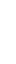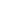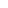# Pong in PixiJS

February 06, 2020

# Creating Pong with PixiJS

PixiJS is a 2D WebGL renderer that uses Canvas2D as a backup. It’s known for its fast performance, and is used for 2D browser games or adding graphics to websites. I’ll be covering the basics of setting up a PixiJS program and implementing a basic game, Pong.

Here is the github repo of the game that we’ll be coding throughout the blog.

https://github.com/DanielOhn/blog_pong

## Setting Up

This can be done in a single html file using `<script>` to insert most of your PixiJS code, but in this tutorial we’ll use seperate files.

In the end, our directory will look similar to this:

``````- Pong Folder
-- index.html
-- index.css
-- app.js
-- images/
---- bar.png
---- ball.png
---- wall.png``````

Let’s get started with creating our root directory, and the main files.

``````mkdir pong && cd pong
touch index.html
touch index.css
touch app.js``````

In the index, we are going to add the latest version of PixiJS, along with the javascript file and the css.

index.html

``````<!DOCTYPE html>
<html>
<meta charset='utf-8' />
<title>PixiJS Pong</title>
<body>
<canvas id='mycanvas'></canvas>
<script src="https://cdnjs.cloudflare.com/ajax/libs/pixi.js/5.1.3/pixi.min.js">
</script>
<script src='app.js'></script>
</body>
</html>``````

In the `CSS` file, we are just gonna make the padding and margin for the body 0.

index.css

``````body {
margin: 0;
}``````

Now we add our `app.js` file and make the program just print out that it’s getting the proper version of PixiJS.

app.js

``````const canvas = document.getElemenyById('mycanvas');

let type = 'WebGL';

if (!PIXI.utils.isWebGLSupported()) {
type = 'canvas';
}

PIXI.utils.sayHello(type);``````

Opening the `index.html` file should show if PixiJS is running properly in the browser, and if we open our console it should say the following:

``PixiJS 5.1.3 - WebGL - http://www.pixijs.com/``

There! We have PixiJS running, now we can begin working on Pong and getting images to display in our app.

## Displaying an Image

Create a new directory called `/images` and place the following three `.png` files within there.

``mkdir images``
• bar.png

•• ball.png

•• wall.png

•Now let’s get our app to get a single image going in the center of the screen. We’ll be loading it in with `PIXI.Loader.shared` and setting up our application in `PIXI.Application`.

app.js

``````const canvas = document.getElemenyById('mycanvas');

const app = new PIXI.Application({
view: canvas,
width: window.innerWidth,
height: window.innerHeight,
});

let image;

function setup() {

image = new PIXI.Sprite(texture);

image.x = app.screen.width / 2;
image.y = app.screen.height / 2;

}

function game(delta) {}``````

To get our image to display and our PixiJS app running properly we need to run a server. We can download http-server and run it in our terminal to get a basic node server running so we can properly use images within our app.

Once we download the program, we can run the server in the root directory by running the following command in the terminal:

``http-server -a localhost -p 3000``

We set it up so we can access our app at `localhost:3000`. Now going to the address we can see that our app is displaying a single bar in the middle of the screen.

## Layout

Now that we can display a single image, we should make a basic layout for everything that we’ll be using in the game. We’ll need a player, an enemy, a top wall, a bottom wall, and the ball.

Let’s display all of those within our code.

``````// Below App Function
let player, enemy, ball;
let floors = [];
let walls = [];

function setup() {

// Creating container to set all our sprites inside.
let stage = new PIXI.Container();

// Placing the stage container in the center of the screen
stage.x = app.screen.width / 2;
stage.y = app.screen.height / 2;

// Adding Player and Enemy Sprites and Positions
player = new PIXI.Sprite(bar_texture);

player.x = -300;
player.anchor.set(.5);

enemy = new PIXI.Sprite(bar_texture);

enemy.x = 300;
enemy.anchor.set(.5);

ball = new PIXI.Sprite(ball_texture);

ball.x = 0;
ball.anchor.set(.5);

// Setting up the walls
for (let i = 0; i < 75; i++) {
let new_wall = new PIXI.Sprite(wall_texture);
let new_floor = new PIXI.Sprite(wall_texture);

walls.push(new_wall);

walls[i].y = -200;
walls[i].x = -300 + i * 8;

walls[i].anchor.set(.5);

floors.push(new_floor);

floors[i].y = 200;
floors[i].x = -300 + i * 8;
floors[i].anchor.set(.5);
}
}``````

By running the server and going to `localhost:3000` we should get the layout of our game Pong on the screen.

## Movement

Let’s get basic movement going with the ball by making it move immediately in any direction when we start up the program.

``````function setup() {
// Previous Code
// We will use this to keep track of the ball's direction
ball.vx = 1;
ball.vy = 0;

// Player and Enemy would just move up and down, so it'll just need vy.
player.vy = 0;
enemy.vy = 0;

}

function game(delta) {
let speed = 5 * delta;

ball.x += ball.vx * speed;
}``````

Now when we open up the `html` file with the server running in the terminal, we can see that the ball moves instantly to a direction.

## Controls

Below our `game` function, we are going to add this `keyboard` function. It’s from kittykatattack’s tutorial on Learning Pixi. Which is a great tutorial if you want to learn about PixiJS, I highly recommend it.

``````function setup() {
// Rest of Code
}

function game(delta) {
// Rest of Code
}

function keyboard(value) {
let key = {};
key.value = value;
key.isDown = false;
key.isUp = true;
key.press = undefined;
key.release = undefined;

key.downHandler = event => {
if (event.key === key.value) {
if (key.isUp && key.press) key.press();
key.isDown = true;
key.isUp = false;
event.preventDefault();
}
};

key.upHandler = event => {
if (event.key === key.value) {
if (key.isDown && key.release) key.release();
key.isDown = false;
key.isUp = true;
event.preventDefault();
}
};

// Attach Event listeners
const downListener = key.downHandler.bind(key);
const upListener = key.upHandler.bind(key);

// Detach event listeners
key.unsubscribe = () => {
window.removeEventListener("keydown", downListener);
window.removeEventListener("keyup", upListener);
};

return key;
};``````

Now we add controls to move the player up and down. We are going to create two new variables outside of the `setup` function called up and down, and assign them keys with our `keyboard` function.

``````let up = keyboard('w');
let down = keyboard('s');

function setup() {
// All previous code in setup

// Controls
up.press = () => {
player.vy = -1;
};

up.release = () => {
player.vy = 0;
};

down.press = () => {
player.vy = 1;
};

down.release = () => {
player.vy = 0;
};

}

function game(delta) {
// Rest of code
}``````

## Collision

We will be adding collision to our small little pong game. Especially since we managed to get the player to move, along with the ball. We could easily implement a function to have the enemy move as well which we will do after adding collision into the game.

Let’s add this function, which is a modified version of collision checking from kittykatattack’s tutorial on PixiJS. I changed the center of the rectangles to account for setting the anchor of each Sprite into it’s center.

Now we will add the collision function below the `game` function.

``````function check_collid(r1, r2) {
// Define variables we'll use to calculate
let hit, combinedHalfWidths, combinedHalfHeights, vx, vy;

// hit will determine whether there's a collision
hit = false;

// Find the center points of each sprite
r1.centerX = r1.x;
r1.centerY = r1.y;

r2.centerX = r2.x;
r2.centerY = r2.y;

// Find the half-widths and half-heights of each sprite
r1.halfWidth = r1.width / 2;
r1.halfHeight = r1.height / 2;
r2.halfWidth = r2.width / 2;
r2.halfHeight = r2.height / 2;

// Calculate the distance vectors between sprites
vx = r1.centerX - r2.centerX;
vy = r1.centerY - r2.centerY;

// Figure out the combined half-widths and half-heights
combinedHalfWidths = r1.halfWidth + r2.halfWidth;
combinedHalfHeights = r1.halfHeight + r2.halfHeight;

// Check collision on x axis
if (Math.abs(vx) < combinedHalfWidths) {
// A collisoin might be occuring.  Check for it on y axis
if (Math.abs(vy) < combinedHalfHeights) {
// There's definitely a collision happening
hit = true;
} else {
hit = false;
}
} else {
hit = false;
}

return hit;
}``````

Now that we have the collision function implemented, we need to do the following to get our game of Pong going:

• Ball bouncing off the Players and Walls
• Player can’t move past walls
• Enemy can’t move past walls

Let’s implement the collision of the ball with the player and enemy. We’ll add the following code to your `game` function:

``````function game(delta) {
let speed = 5 * delta;

// Check Collision of Ball with Player + Enemy
if (check_collid(ball, enemy) || check_collid(ball, player)) {
ball.vx *= -1;
}

// Movement for ball and players
ball.x += ball.vx * speed;
ball.y += ball.vy * speed;

player.y += player.vy * speed;
enemy.y += enemy.vy * speed;
}``````

Now when we run the program, we can see that our ball bounces back and forth between the two. Very cooL!

Now let’s implement the collision on both the ball and walls. We’ll run two for-of loops to iterate through all our walls and floors, then checking if the ball collids with any.

``````function setup() {
ball.vx = 0;
ball.vy = 1;
}

function game(delta) {
let speed = 5 * delta;

for (let wall of walls) {
if (check_collid(ball, wall)) {
ball.vy = 1;
}
}

for (let floor of floors) {
if (check_collid(ball, floor)) {
ball.vy = -1;
}
}
// Other code
}``````

Bam! Now if we run the program, it’ll just make the ball bounce up and down between the two walls.

Now we just need to implement the collision between the players and the walls. We’ll just prevent the enemy and player from being able to move past the walls confining them into the small space.

``````function game(delta) {
let speed = 5 * delta;

// Check Collision of Walls
for (let wall of walls) {
if (check_collid(player, wall)) {
if (player.vy < 0) {
player.vy = 0;
}
}

if (check_collid(enemy, wall)) {
if (enemy.vy < 0) {
enemy.vy = 0;
}
}

if (check_collid(ball, wall)) {
ball.vy = 1;
}
}

for (let floor of floors) {
if (check_collid(player, floor)) {
if (player.vy > 0) {
player.vy = 0;
}
}

if (check_collid(enemy, floor)) {
if (enemy.vy > 0) {
enemy.vy = 0;
}
}

if (check_collid(ball, floor)) {
ball.vy = -1;
}
}

// Other code
}``````

Now if we move our player up and down we can see that it is stopped right when it crashes into the walls for both sides. With that, it should cover all the collision needed for the game and we can move on to the game logic.

## Game Logic

For this section, we’ll be adding the following:

• Basic AI for Enemy
• Reseting Ball

Once we add these components, we’ll be completely finished with our main logic for the game.

Let’s have the enemy just following the ball around based on it’s Y Coordinate. We’ll add it in our `game` function, since we want it to consistently check and move the enemy based on the ball’s position.

``````function game(setup) {
let speed = 5 * delta;

// Comparing Y coordinates of enemy and ball
// makes the enemy follow ball around
if (ball.y > enemy.y) {
enemy.vy = 1;
} else if (ball.y < enemy.y) {
enemy.vy = -1;
} else {
enemy.vy = 0;
}

// Rest of Code
}``````

We have the enemy following around the ball as it shifts up and down, but we need to have the ball moving about more and have it be able to go out of bounds before we attempt to reset it.

``````function setup() {
// All Code Above

ball.vx = 1;
ball.vy = 1;

// All Code Below
}``````

With this simple change, we could see the ball moving about diagnoal and bouncing around the place. It forces both the player and enemy to move about to hit the ball back and forth. However, the enemy will always be able to hit the ball with it’s current speed.

``````function game(delta) {
let speed = 3 * delta;
let ball_speed = 5 * delta;

// Collision Code and whatnot
ball.x += ball.vx * ball_speed;
ball.y += ball.vy * ball_speed;

player.y += player.vy * speed;
enemy.y += enemy.vy * speed;
}``````

By changing lowering the speed, the ball can now move faster than the AI at times and could slip past it if it bounces properly. Time to reset the ball if it goes out of bounds.

We’ll look at the position of our player and enemy and see that they are -300 and 300 for the X Coordinate. If the ball exceeds or dips below these values then we know that it should be reset to the center.

``````// Places ball in center of screen, and changes its direction
function ball_reset() {
ball.x = 0;
ball.y = 0;
ball.vy = ball.vy * -1;
ball.vx = ball.vx * -1;
}

function game(setup) {
// speeds

// If ball goes out of bounds, reset it.
if (ball.x > 325) {
ball_reset();
} else if (ball.x < -325) {
ball_reset();
}

// Other code
}``````

Great! We mananged to get a simple game of Pong finished in PixiJS, all we need to add now is the score.

## Score

In this section, we will do the following:

• Setting Text Style
• Implementing Text
• Iterating Scores

In the `setup`, right above the controls for up/down let’s add the text style and add basic text to the stage. We’ll need to make sure to add two variables above the `setup` function when we initialize all the other variables.

``````// Add Scores to this line
let player, enemy, ball, playerScore, enemyScore;

function setup() {
// ! CODE !

// Size, Color, and Font of the text we are adding
const style = new PIXI.TextStyle({
fontFamily: 'Roboto',
fill: ['#ffffff'],
fontSize: 32,
});

// Adding Score to our Player and Enemy Object
player.score = 0;
enemy.score = 0;

// Creating the actual Text for the scores.
playerScore = new PIXI.Text(player.score, style);
enemyScore = new PIXI.Text(enemy.score, style);

// Controls Below
}``````

With this we should be able to see a large, white 0 in the middle of the screen. Both of these scores are overlapping, so we need to position them above the top wall in their appropriate places.

``````function setup() {
playerScore.x = -275;
playerScore.y = -250;

enemyScore.x = 250  ;
enemyScore.y = -250;

// Controls Below
}``````

Our scores are displaying in the right areas, right above the wall ontop and in the right places to represent the player/enemy score.

Now we need to iterate the appropriate score whenever a ball goes out of bounds.

``````function game(delta) {
//speeds

if (ball.x > 325) {
ball_reset();
player.score++;
playerScore.text = player.score;
} else if (ball.x < -325) {
ball_reset();
enemy.score++;
enemyScore.text = enemy.score;
}

// other code
}``````

Playing the game, we can see that the score updates itself properly with the correct score.

That’s all! We managed to completely code a game of Pong in PixiJS, even though there are a few bugs in it. I hope this helps someone get a better understanding of using PixiJS, and if you want to leave feedback or find any issues with this blog feel free to contact me on Twitter @ohndaniel_.